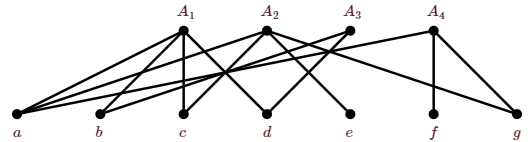# 4.5: Introduction to Graph Theory

$$\newcommand{\vecs}{\overset { \rightharpoonup} {\mathbf{#1}} }$$ $$\newcommand{\vecd}{\overset{-\!-\!\rightharpoonup}{\vphantom{a}\smash {#1}}}$$$$\newcommand{\id}{\mathrm{id}}$$ $$\newcommand{\Span}{\mathrm{span}}$$ $$\newcommand{\kernel}{\mathrm{null}\,}$$ $$\newcommand{\range}{\mathrm{range}\,}$$ $$\newcommand{\RealPart}{\mathrm{Re}}$$ $$\newcommand{\ImaginaryPart}{\mathrm{Im}}$$ $$\newcommand{\Argument}{\mathrm{Arg}}$$ $$\newcommand{\norm}{\| #1 \|}$$ $$\newcommand{\inner}{\langle #1, #2 \rangle}$$ $$\newcommand{\Span}{\mathrm{span}}$$ $$\newcommand{\id}{\mathrm{id}}$$ $$\newcommand{\Span}{\mathrm{span}}$$ $$\newcommand{\kernel}{\mathrm{null}\,}$$ $$\newcommand{\range}{\mathrm{range}\,}$$ $$\newcommand{\RealPart}{\mathrm{Re}}$$ $$\newcommand{\ImaginaryPart}{\mathrm{Im}}$$ $$\newcommand{\Argument}{\mathrm{Arg}}$$ $$\newcommand{\norm}{\| #1 \|}$$ $$\newcommand{\inner}{\langle #1, #2 \rangle}$$ $$\newcommand{\Span}{\mathrm{span}}$$$$\newcommand{\AA}{\unicode[.8,0]{x212B}}$$

We can interpret the sdr problem as a problem about graphs. Given sets $$A_1,A_2,\ldots,A_n$$, with $$\bigcup_{i=1}^n A_i=\{x_1,x_2,\ldots,x_m\}$$, we define a graph with $$n+m$$ vertices as follows: The vertices are labeled $$\{A_1,A_2,\ldots,A_n,x_1,x_2,\ldots x_m\}$$, and the edges are $$\{\{A_i,x_j\}\mid x_j\in A_i\}$$.

##### Example $$\PageIndex{1}$$

Let $$A_1=\{a,b,c,d\}$$, $$A_2=\{a,c,e,g\}$$, $$A_3=\{b,d\}$$, and $$A_4=\{a,f,g\}$$. The corresponding graph is shown in Figure $$\PageIndex{1}$$.Figure $$\PageIndex{1}$$: A set system depicted as a bipartite graph.

Before exploring this idea, we introduce a few basic concepts about graphs. If two vertices in a graph are connected by an edge, we say the vertices are adjacent. If a vertex $$v$$ is an endpoint of edge $$e$$, we say they are incident. The set of vertices adjacent to $$v$$ is called the neighborhood of $$v$$, denoted $$N(v)$$. This is sometimes called the open neighborhood of $$v$$ to distinguish it from the closed neighborhood of $$v$$, $$N[v]=N(v)\cup\{v\}$$. The degree of a vertex $$v$$ is the number of edges incident with $$v$$; it is denoted $$\text{d}(v)$$.

Some simple types of graph come up often: A path is a graph $$P_n$$ on vertices $$v_1,v_2,\ldots,v_n$$, with edges $$\{v_i,v_{i+1}\}$$ for $$1\le i\le n-1$$, and no other edges. A cycle is a graph $$C_n$$ on vertices $$v_1,v_2,\ldots,v_n$$ with edges $$\{v_i,v_{1+(i\bmod n)}\}$$ for $$1\le i\le n$$, and no other edges; this is a path in which the first and last vertices have been joined by an edge. (Generally, we require that a cycle have at least three vertices. If it has two, then the two are joined by two distinct edges; when a graph has more than one edge with the same endpoints it is called a multigraph. If a cycle has one vertex, there is an edge, called a loop, in which a single vertex serves as both endpoints.) The length of a path or cycle is the number of edges in the graph. For example, $$P_1$$ has length 0, $$C_1$$ has length 1. A complete graph $$K_n$$ is a graph on $$v_1,v_2,\ldots,v_n$$ in which every two distinct vertices are joined by an edge. See Figure $$\PageIndex{2}$$ for examples.Figure $$\PageIndex{2}$$: Graphs $$P_5$$, $$C_6$$, $$K_5$$.

The graph in Figure $$\PageIndex{1}$$ is a bipartite graph.

##### Definition $$\PageIndex{1}$$: Bipartite

A graph $$G$$ is bipartite if its vertices can be partitioned into two parts, say $$\{v_1,v_2,\ldots,v_n\}$$ and $$\{w_1,w_2,\ldots,w_m\}$$ so that all edges join some $$v_i$$ to some $$w_j$$; no two vertices $$v_i$$ and $$v_j$$ are adjacent, nor are any vertices $$w_i$$ and $$w_j$$.

The graph in Figure $$\PageIndex{1}$$ is bipartite, as are the first two graphs in Figure $$\PageIndex{2}$$.

This page titled 4.5: Introduction to Graph Theory is shared under a CC BY-NC-SA 3.0 license and was authored, remixed, and/or curated by David Guichard via source content that was edited to the style and standards of the LibreTexts platform; a detailed edit history is available upon request.